Related Articles

# Hypergraph & its representation | Discrete Mathematics

• Last Updated : 29 Jun, 2021

Introduction :
A hypergraph is a graph in which hyperedges (generalized edges) can connect to a subset of vertices/nodes rather than two vertices/nodes.
The edges (also known as hyperedges) of a hypergraph are arbitrary nonempty sets of vertices. A k-hypergraph has all such hyperedges connecting exactly k vertices; a normal graph is thus a 2-hypergraph (as one edge connects 2 vertices).

Hypergraph representation :
An undirected hypergraph H is defined as a pair H = (V,E), where V is a set of items known as nodes or vertices, and E is a set of non-empty subsets of V, known as hyperedges or edges (in an undirected hypergraph).
Here, E is a subset of P(X), where P(X) is the Power Set of X.
Each hyperedge can be represented as a closed curve containing its members to create hypergraphs.
Example –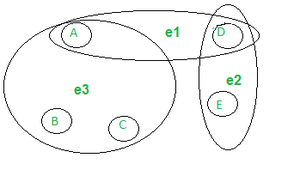Hypergraph H

H(V) = { A, B, C, D, E}
H(E) = {e1, e2, e3 } = { {A, D}, {D, E}, {A, B, C} }

Order & Size Of Hypergraph :
The order of the hypergraph = the size of the vertex set, and
The size of the hypergraph = the size of the edges set.

```Order(H) = |H(V)|
Size(H)= |H(E)|```

The above hypergraph has –
Order(H) = |H(V)| = 5
Size(H)= |H(E)| = 3

Hypergraph To Bi-Partite Graph :
Because it is always possible (though not always convenient) to express a hypergraph by a bipartite graph, hypergraphs are rarely utilized. The vertex set in a bipartite graph can be divided into two subsets, P and Q, with each edge connecting a vertex in P to a vertex in Q.
We simply represent the vertices of H as vertices in Q and the hyperedges of H as vertices in P, and insert an edge (p, q) whenever s is a member of the hyperedge t in H.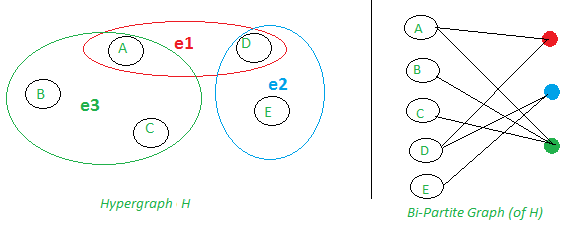A hypergraph is depicted in two ways. Five vertices on the left are connected by three hyperedges. On the right, the same five vertices are joined to new vertices(three) representing the hyperedges by ordinary edges.

Properties Of Hypergraph :
A hypergraph can have a number of different properties, including –

• Empty Hypergraph –
There are no edges in the empty hypergraph. As you see, the figure below has no edges but 5 vertices named : A B, C, D, E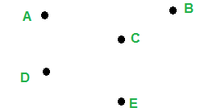Empty Hypergraph

• d – Regular –
Every vertex has a degree of d, implying that it is contained in precisely d hyperedges.
Example : Below, the hypergraph is 2- regular because all the vertices (A, B & C) have the same degree : 2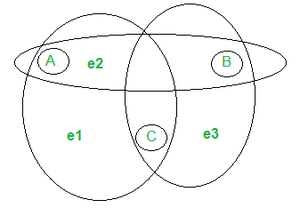2- regular hypergraph

• 2-colorable –
Its vertices can be divided into two classes, P and Q, so that each hyperedge with a cardinality of at least 2 has at least one vertex from each class.
• Non – Simple –
Has loops (hyperedges with a single vertex) or repeating edges (two or more edges with the same set of vertices)
Example –
In the graph below, we can see 2 loops : e1 & e2e, so it is a non-simple hypergraph.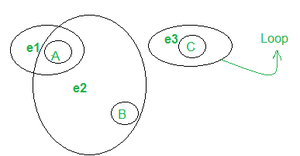Non – SImple HyperGraph

• Simple –
There are no loops or repeating edges in this design.
• k -uniform –
Each hyperedge is made up of exactly k vertices.
Example –
In the below hypegraph we can see that each hyperedge (e1 , e2, e3, e4) consists of 2 vertices, therefore it’s a 2- uniform hypergraph.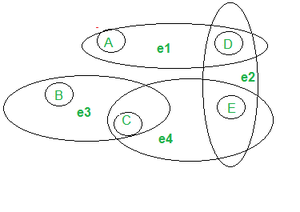2-Uniform HyperGraph

• k -partite –
Each hyperedge comprises exactly one vertex of each type, and the vertices are divided into k parts.
Example –
In the hypergraph below, say,  the vertices are partitioned into 3 parts :m (A, D),(B,E) & (D,F) . Note that each hyperedge contains only one vertex of each partition.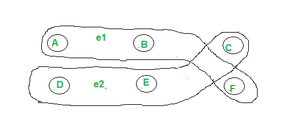3-partite hypergraph

Attention reader! Don’t stop learning now. Get hold of all the important CS Theory concepts for SDE interviews with the CS Theory Course at a student-friendly price and become industry ready.

My Personal Notes arrow_drop_up